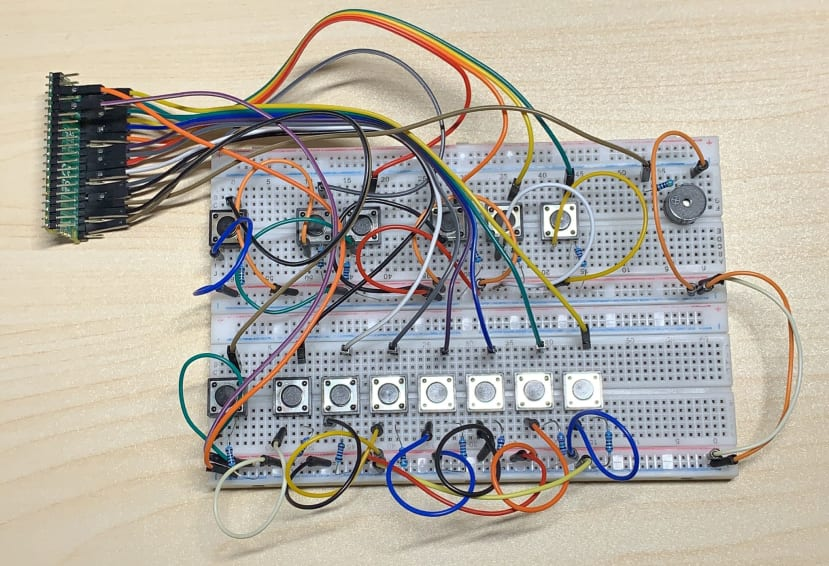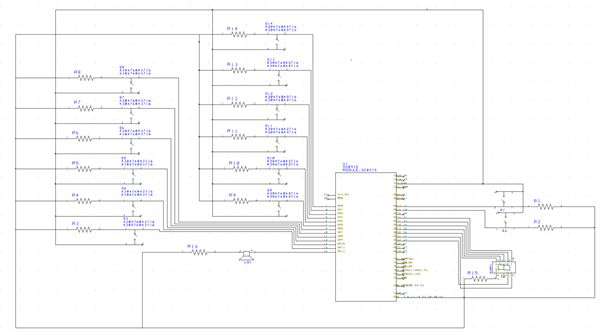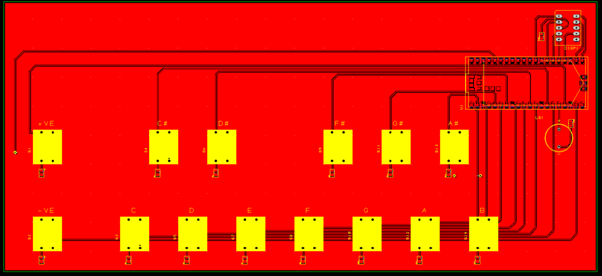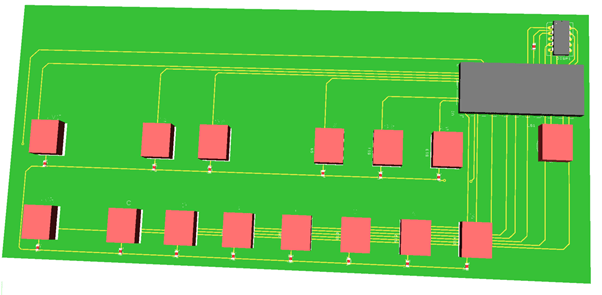# Raspberry Pi Pico Piano – Part 1

### Do you have enough space to place an 88-keys piano?Today I want to share my newly designed 88-key piano with only 14 buttons.

Most musical instruments are portable, but have you ever thought about taking your piano to a picnic or even camping? You might say it's impossible. There's no way to get that giant instrument outside, even if it's just for class. But I suppose you might be wrong this time, and I made a portable piano with complete 88-keys piano by using a Raspberry Pi Pico .

There are many foldable digital pianos in the market, and some can fold one time, some can fold two times, but they are still cumbersome. In my design, I will only place one octave(12-buttons) on the circuit and use two buttons to adjust the octave.

I tried to use DesignSpark PCB to draw the schematic diagram and translate it to PCB, it worked very well. But there are some components that the DesignSpark library doesn't have, then I went to SnapEDA to download and it was easy to import into DesignSpark PCB.

#### PCB DesignSchematic diagram. aPCB3D View

DesignSpark PCB also allows me to view the PCB in 3D view after I have finished routing the PCB, which I think is a wonderful feature, sometimes I may not print the PCB, but it also allows me to see the final product in 3D.

#### Software Part

Firstly, we have pitches.h files to define each note of the frequency.

Then, we have 2 buttons to change the octave, and a 7-segment to display which octaves are playing. Also, there have 12 buttons that have each note.

A resistor is linked to a buzzer to pin 12.

The duration of each note is 100ms.

``````const int c = 11;
const int cs = 10;
const int d = 9;
const int ds = 8;
const int e = 7;
const int f = 6;
const int fs = 5;
const int g = 4;
const int gs = 3;
const int a = 2;
const int as = 1;
const int b = 0;

const int Buzz = 12;

const int up = 13;
const int down = 14;

int up_State = 0;
int down_State = 0;

int count_value =0;
int prestate =0;

int t=100; // ...for 0.1 sec
``````

pinMode is set the Arduino digital pin as output or input

``````void setup() {
// initialize the pushbutton pin as an input:
pinMode(up, INPUT);
pinMode(down, INPUT);
//pinMode(buttonPin3, INPUT);

pinMode(LED, OUTPUT);

pinMode(c, INPUT);
digitalWrite(c,HIGH);

pinMode(cs, INPUT);
digitalWrite(cs,HIGH);

pinMode(d, INPUT);
digitalWrite(d,HIGH);

pinMode(ds, INPUT);
digitalWrite(ds,HIGH);

pinMode(e, INPUT);
digitalWrite(e,HIGH);

pinMode(f, INPUT);
digitalWrite(f,HIGH);

pinMode(fs, INPUT);
digitalWrite(fs,HIGH);

pinMode(g, INPUT);
digitalWrite(g,HIGH);

pinMode(gs, INPUT);
digitalWrite(gs,HIGH);

pinMode(a, INPUT);
digitalWrite(a,HIGH);

pinMode(as, INPUT);
digitalWrite(as,HIGH);

pinMode(b, INPUT);
digitalWrite(b,HIGH);

digitalWrite(LED,LOW);
}
``````

There are 9 octaves for the piano, which is the same as the real piano. And each octave, I used a void loop to contain it.

``````void count()
{
// check if the pushbutton is pressed. If it is, the buttonState is HIGH:
if (up_State == HIGH && prestate == 0) {
count_value++;
Serial.print(count_value);
prestate = 1;
}
//decrement
else if (down_State == HIGH && prestate == 0) {
count_value--;
Serial.print(count_value);
prestate = 1;
}
else if(up_State == LOW && down_State == LOW) {
prestate = 0;
}
}

void one()
{
digitalWrite(16,LOW);   //A
digitalWrite(17,HIGH);  //B
digitalWrite(18,HIGH);  //C
digitalWrite(19,LOW);   //D
digitalWrite(20,LOW);   //E
digitalWrite(21,LOW);   //F
digitalWrite(22,LOW);   //G
}

void piano1()
{
{
tone(Buzz,T_a1);
digitalWrite(LED,HIGH);
delay(t);        //time
noTone(Buzz);
}

{
tone(Buzz,T_as1);
digitalWrite(LED,HIGH);
delay(t);        //time
noTone(Buzz);
}

{
tone(Buzz,T_b1);
digitalWrite(LED,HIGH);
delay(t);        //time
noTone(Buzz);
}
else{
noTone(Buzz);
digitalWrite(LED,LOW);
}
}
``````

By pressing the change octave button, which is a counter to switch the octave of the piano, and which will show the octave number on the seven segments. Also, there has a LED linked to pin 15, then press the 12 buttons of the piano, which will also light up.

``````void loop() {
// read the state of the pushbutton value:

count();

if (count_value == 1)
{
Serial.print("1");
piano1();
}
else if (count_value == 2)
{
Serial.print("2");
piano2();
}
else if (count_value == 3)
{
Serial.print("3");
piano3();
}
else if (count_value == 4)
{
Serial.print("4");
piano4();
}
else if (count_value == 5)
{
Serial.print("5");
piano5();
}
else if (count_value == 6)
{
Serial.print("6");
piano6();
}
else if (count_value == 7)
{
Serial.print("7");
piano7();
}
else if (count_value == 8)
{
Serial.print("8");
piano8();
}
else if (count_value == 9)
{
Serial.print("9");
piano9();
}
else
{
Serial.print(" ");
}
}
``````

#### Future Development

At this stage, the piano can only press one note at a time. In Part 2, I will build a piano that can press multiple notes at the same time.